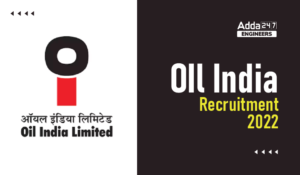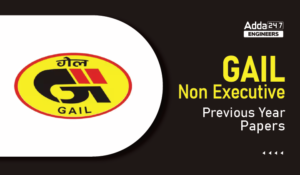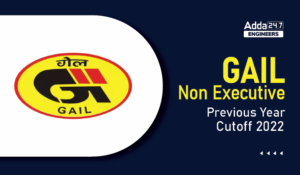Engineering Jobs   »   QUIZ: CIVIL ENGINEERING( 10-july-2020)

# QUIZ: CIVIL ENGINEERING( 10-july-2020)

Quiz: Civil Engineering
Exam: UKSSSC-JE
Topic: miscellaneous

Each question carries 1 mark
Negative marking: 1/4 mark
Time: 10 Minutes

Q1. Francis turbine is a:
(a) Axial flow turbine
(c) impulse turbine
(d) Outward flow turbine

Q2. In reaction turbine-
(a) flow can be regulated without loss
(b) water may be allowed to enter apart of whole of wheel circumference
(c) the outlet must be above the tail race
(d) there is only partial conversion of available head to velocity head before entry to runner

Q3. Kaplan turbine-
(b) is used where high head is available
(c) has inlet adjusted guided vanes

Q4. Weber number is the ratio of inertial force to
(a) gravitational force
(b) surface tension
(c) elasticity
(d) viscosity

Q5. A Pelton wheel is:
(b) Inward flow impulse turbine
(c) Axial flow impulse turbine
(d) Outward flow impulse turbine

Q6. Maximum theoretical efficiency of Pelton wheel is obtained when the ratio of bucket speed to jet speed is:
(a) 0.26
(b) 0.98
(c) 0.46
(d) 0.58

Q7. If E_c and E_s are modulus of elasticity of concrete and steel respectively, then the modular ratio (m) will be
(a) E_c/E_s
(b) E_s/E_c
(c) (E_c+E_s)/(E_s-E_c )
(d) (4E_c)/E_s

Q8.Partial safety factors for concrete and steel respectively may be taken as:
(a) 1.5 and 1.15
(b) 1.5 and 1.78
(c) 3 and 1.78
(d) 3 and 1.2

Q9. The ratio of the maximum diameter of the reinforcing bar in a slab and the total thickness of the slab should not exceed …………….
(a) 1/4
(b) 1/5
(c) 1/6
(d) 1/8

Q10. A sand deposit has a porosity of 1/3 and its specific gravity is 2.5. The critical hydraulic gradient to cause sand boiling in the stratum will be
(a) 1.5
(b) 1.25
(c) 1.0
(d) 0.75

SOLUTION

S1. Ans.(b)
Sol. Francis turbine is inward flow radial reaction turbine.

S2. Ans.(d)
Sol. in a reaction turbine, there is only partial conversion of available heat to velocity head before entry to runner. Thus at entrance to reaction turbine the fluid will have both pressure energy as well as kinetic energy.

S3. Ans.(d)

S4. Ans.(b)
Sol. Weber number is the ratio of intertie force to surface tension.

S5. Ans.(b)
Sol. Pelton wheel is the turbine comes in category of inward flow impulse turbine.

S6. Ans.(c)
Sol. maximum actual efficiency.
When
V = 2u
u = 0.5 V
but,
Theoretically maximum efficiency.
▭(u=0.46V)

S7. Ans.(b)
Sol. ▭(Modular Ratio (m)=(Modulas of elasticity of steel(E_s ))/(Modulus of plasticity of concrete(E_c ) ))
E_s=2×10^5 N\/mm^2
E_c=5000 √fck (Acc. To IS 456:2000)

S8. Ans.(a)
Sol. In limit state method.
Partial safety factor for RCC = 1.5
Partial safety factor for steel = 1.15
In working state method.
Partial safety factor for R.C.C. = 3
Partial safety factor for steel = 1.78

S9. Ans.(d)
Sol. ▭(maximum diameter of reinforcement in slab ≯1/8× thickness of slab)
S10. Ans.(c)
Sol. porosity (n) = 1/3
G = 2.5
Critical hydraulic gradient (ic) = ?
e = n/(1-n )=(1\/3)/(2\/3)=0.5
▭(e=0.5)
i_c=(G-1)/(1+e)=(2.5-1)/(1+0.5)=1.5/1.5
▭(i_c=1 )

Sharing is caring!

Thank You, Your details have been submitted we will get back to you.
•DVC Interview Schedule 2022, Download DV...
•ONGC Non Executive Answer Key 2022, Down...
•Oil India Recruitment 2022, Apply Online...
•GAIL Non Executive Previous Year Papers,...
•GAIL Non Executive Previous Year Cutoff ...
•APWD Previous Year Papers, Check here fo...